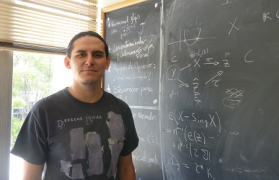## 25/01/2022, Tuesday, 16:30–17:30 Europe/Lisbon OnlineGiancarlo Urzua, Pontificia Universidad Católica de Chile
What is the right combinatorics for spheres in K3 surfaces?

Together with Javier Reyes, in https://arxiv.org/abs/2110.10629 we have been able to construct compact 4-manifolds $3\mathbb{CP}^2\#(19-K^2)\overline{\mathbb{CP}}^2$ with complex structures for $K^2=1,2,3,4,5,6,7,8,9$. The cases $K^2=7,9$ are completely new in the literature, and this finishes with the whole range allowed by the technique of Q-Gorenstein smoothing (rational blow-down). But one can go further: Is it possible to find minimal exotic $3\mathbb{CP}^2\#(19-K^2)\overline{\mathbb{CP}}^2$ for $K^2\geq10$? Here it would be much harder to prove the existence of complex structures, but, as a motivation, there is not even one example for $K^2 > 15$, and very few for $10 \leq K^2 \leq 15$ (see e.g. works by Akhmedov, Park, Baykur). In this talk I will explain the constructions in connection with the geography of spheres arrangements in $K3$ surfaces, where the question of the title arises. We do not have an answer. So far we have been implementing what we know in computer searches, finding these very rare exotic surfaces for $K^2=10,11,12$. This is a new and huge world which promises more findings, we have explored very little.